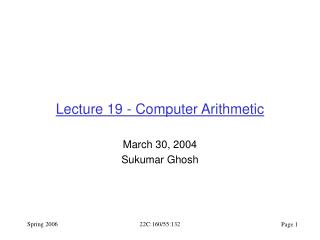Download PresentationLecture 19 - Computer Arithmetic

# Lecture 19 - Computer Arithmetic

Download Presentation## Lecture 19 - Computer Arithmetic

- - - - - - - - - - - - - - - - - - - - - - - - - - - E N D - - - - - - - - - - - - - - - - - - - - - - - - - - -
##### Presentation Transcript

1. Lecture 19 - Computer Arithmetic March 30, 2004 Sukumar Ghosh 22C:160/55:132

2. Carry-ripple Adder • Each cell: ri = aiXOR biXOR cin cout = aicin + aibi + bicin = cin(ai + bi) + aibi • 4-bit adder: “Full adder cell” 22C:160/55:132

3. Adders (cont.) Ripple Adder Ripple adder is inherently slow because, in general s7 must wait for c7 which must wait for c6 … Tan, Costan How do we make it faster, perhaps with more cost? 22C:160/55:132

4. Carry Select Adder T = Tripple_adder / 2 + TMUX 22C:160/55:132

5. Carry Select Adder • Extending Carry-select to multiple blocks • What is the optimal # of blocks and # of bits/block? • If # blocks too large delay dominated by total mux delay • If # blocks too small delay dominated by adder delay T a sqrt(N), Cost 2*ripple + muxes 22C:160/55:132

6. Carry Select Adder • Ttotal = sqrt(N) TFA • assuming TFA = TMUX • For ripple adder Ttotal = N TFA • Is sqrt(N) really the optimum? • From right to left increase size of each block to better match delays • Ex: 64-bit adder, use block sizes [13 12 11 10 9 8 7] • How about recursively defined carry select? 22C:160/55:132

7. a b ci ci+1 s Carry Look-ahead Adders • For n-bit addition best we can achieve is delay  log(n) • How do we arrange this? (think trees) • First, reformulate basic adder stage: carry “propagate” pi = ai bi carry “generate” gi = ai bi ci+1 = gi + pici si = pi ci 22C:160/55:132

8. s0 = p0 c0 c1 = g0 + p0c0 s2 = p2 c2 c3 = g2 + p2c2 s0 = p1 c1 c2 = g1 + p1c1 s3 = p3 c3 c4 = g3 + p3c3 p0 p1 p3 p2 a3 a2 a0 a1 s0 s3 s2 s1 g2 g1 g0 g3 b0 b1 b2 b3 c0 c4 Carry Look-ahead Adders • Ripple adder using p and g signals: • So far, no advantage over ripple adder: T  N 22C:160/55:132

9. Carry Look-ahead Adders • Expand carries: c0 c1 = g0 + p0 c0 c2 = g1 + p1c1 = g1 + p1g0 + p1p0c0 c3 = g2 + p2c2 = g2 + p2g1 + p1p2g0 + p2p1p0c0 c4 = g3 + p3c3 = g3 + p3g2 + p3p2g1 + . . . . . . • Why not implement these equations directly to avoid ripple delay? • Lots of gates. Redundancies (full tree for each). • Gate with high # of inputs. • Let’s reorganize the equations. 22C:160/55:132

10. cin pi pi+1 pi+k gi gi+1 gi+k Carry Look-ahead Adders • “Group” propagate and generate signals: • P true if the group as a whole propagates a carry to cout • G true if the group as a whole generates a carry • Group P and G can be generated hierarchically. P = pi pi+1 … pi+k G = gi+k + pi+kgi+k-1 + … + (pi+1pi+2 … pi+k)gi cout Cout = G + PCin 22C:160/55:132

11. Carry Look-ahead Adders c0 9-bit Example of hierarchically generated P and G signals: a0 Pa b0 a1 a b1 a2 Ga P = PaPbPc b2 c3 = Ga + Pac0 a3 Pb b3 a4 b b4 a5 Gb b5 c6 = Gb + Pbc3 a6 G = Gc + PcGb + PbPcGa Pc b6 a7 c b7 a8 c9 = G + Pc0 Gc b8 22C:160/55:132

12. c0 c4 c8 c0 8-bit Carry Look-ahead Adder with 2-input gates. p0 P8=p0p1 g0 s0 c1= g0+p0c0 G8=g1+p1g0 p1 Pc=P8P9 g1 s1 c2=G8+P8c0 Gc=G9+P9G8 c2 p1 P9=p2p3 g2 c4=Gc+Pcc0 s2 Pe=PcPd c3= g2+p2c2 G9=g3+p3g2 p3 g3 Ge=Gd+PdGc s3 c4 c8=Ge+Pec0 Pa=p4p5 p4 g4 s4 c5= g4+p4c4 Ga=g5+p5g4 p5 Pd=PaPb g5 c6=Ga+Pac4 s5 Gd=Gb+PbGa c6 Pb=p6p7 p6 g6 s6 c7= g6+p6c6 Gb=g7+p7g6 p7 g7 s7 22C:160/55:132

13. Multiplication A = a7 a6 a5 a4 a3 a2 a1 B = b7 b6 b5 b4 b3 b2 b1 A x B = 26.b7.A + 25.b7.A + 24.b7.A + 23.b7.A + … + b7.A Use Carry Save Adders What is CSA? 22C:160/55:132

14. Carry Save Adder 1 1 0 0 1 1 0 1 1 1 0 1 0 1 1 0 0 1 Sum 1 1 0 1 1 1 1 1 0 0 1 Carry computed in parallel 0 Ordinary addition 1 1 0 1 0 0 1 CSA 22C:160/55:132